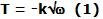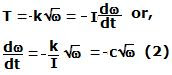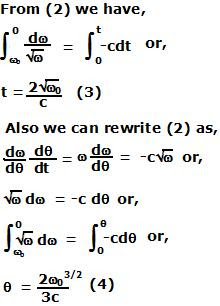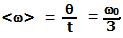## Sunday, November 16, 2008

### Irodov Problem 1.250

Since the torque (moment) due to all forces acting on the flywheel is proportional to the square root of its angular velocity, let this moment be,where k is some constant. Let the moment of inertia of this flywheel be I. Then we have,here c is another constant. The average angular velocity is the total angle the flywheel turned before its topped divided by the total time it took to stop. So we need to compute both these values to compute the average angular velocity.Now the average angular velocity can be computed from (3) and (4) as,